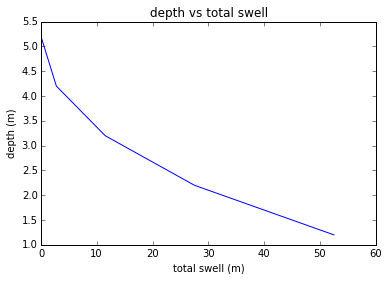# Chapter13:Foundations on Difficult Soils¶

## Ex13.1:Pg-653¶

In :
#example 13.1

Sw=1;
Z=2; # in m
deltaSf=0.0033*Z*Sw*1000; # in mm
print deltaSf,"free surface swell in mm"

6.6 free surface swell in mm


## Ex13.2:Pg-13.2¶

In :
#example 13.2

#from figure 13.11
import matplotlib.pyplot as plt
%matplotlib inline
import numpy
deltaS=1/100.0*1/2.0*(0.55+0+0.55+1.2+1.2+2+2+3);
print deltaS*1000,"total swell in mm"
#partb
D=numpy.array([5.2, 4.2, 3.2, 2.2, 1.2]);
deltaS=numpy.array([0, 0.00275, 0.0115, 0.0275, 0.0525]);
print "depth(m)\t  total swell (m) \n"
for i in range (0,5):
print D[i],"\t            ",deltaS[i]," \n",

plt.plot(deltaS*1000,D);
plt.title("depth vs total swell")
plt.xlabel("total swell (m)")
plt.ylabel("depth (m)")
plt.show()

52.5 total swell in mm
depth(m)	  total swell (m)

5.2 	             0.0
4.2 	             0.00275
3.2 	             0.0115
2.2 	             0.0275
1.2 	             0.0525## Ex13.3:Pg-664¶

In :
#example 13.3

import math
from scipy.optimize import fsolve
phi=12*math.pi/180;
Ds=0.8; # in m
Z=5; # in m
sigmaT=450;
U=math.pi*Ds*Z*sigmaT*math.tan(phi); # in kN
def f(D):
return 1202-450*6.14/1.25*3.14/4*(D**2-0.8**2)
[x]=fsolve(f,1);
Db=x; # in m
print round(Db,2),"diameter of bell in m"
#partb
D=600; # in kN
cu=450; # in kN/m^2
Nc=6.14;
FS=cu*Nc*math.pi/4*(Db**2-Ds**2)/(U-D);
if FS>2 :
print "the structure is compatible with safety measures"

#check bearing capacity

1.15 diameter of bell in m